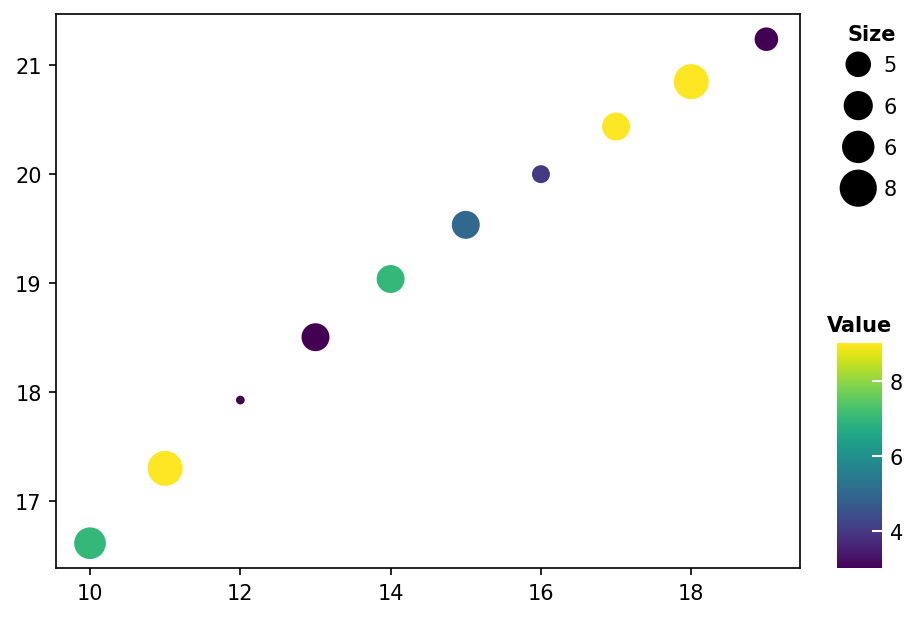# Bubble plot Example#

Here shows how to draw dot plot

```import numpy as np
import milkviz as mv
```

## First let’s create some random data#

```np.random.seed(0)
x = np.arange(10, 20)
y = np.log2(x) * 5
size = np.random.randint(3, 10, 10)
color = np.random.randint(3, 10, 10)
```

## Create the dot heatmap#

```mv.bubble(x=x, y=y, size=size, hue=color,
legend_kw={"title": "Size"}, cbar_kw={"title": "Value"})
``````<AxesSubplot: >
```

Total running time of the script: ( 0 minutes 0.533 seconds)

Gallery generated by Sphinx-Gallery